#2019 iT 邦幫忙鐵人賽DAY 15
0
AI & Data

• 啟用
• 資料匯入
• 資料讀取

## 啟用

postgres=# CREATE EXTENSION postgis;

## 資料匯入

``````import geopandas as gpd

rail.crs = {'init' :'epsg:3826'}
rail=rail.to_crs(epsg=4326)
``````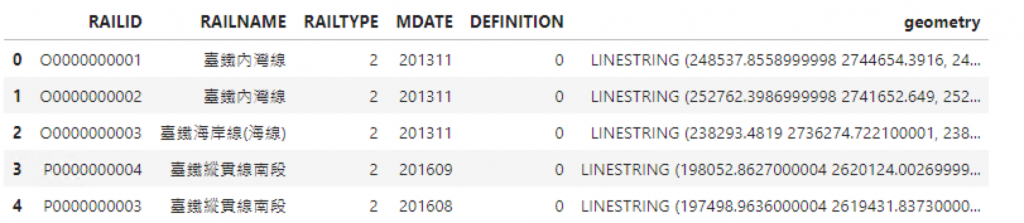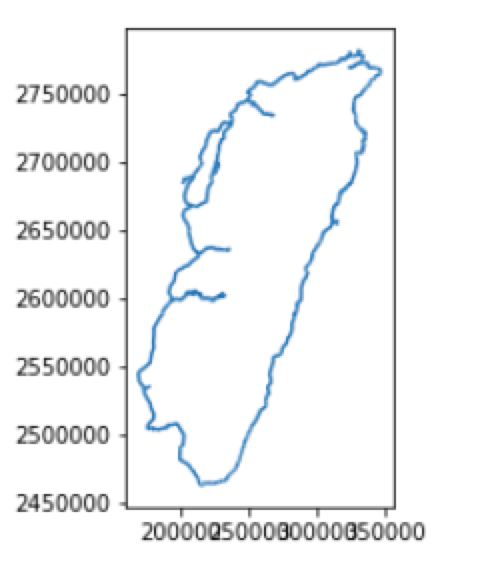``````from sqlalchemy import create_engine
engine = create_engine('postgresql://postgres:postgres@localhost:5432/postgres')
``````

``````from geoalchemy2 import Geometry, WKTElement
rail['geom'] = rail['geometry'].apply(lambda x: WKTElement(x.wkt, srid=4326))
rail.drop('geometry', 1, inplace=True)

``````

``````
rail.to_sql('rail', engine, if_exists='replace', index=False, schema='public',
dtype={'geom': Geometry('LINESTRING', srid= 4326)})
``````

## 資料讀取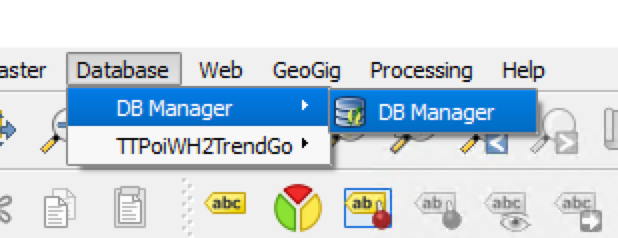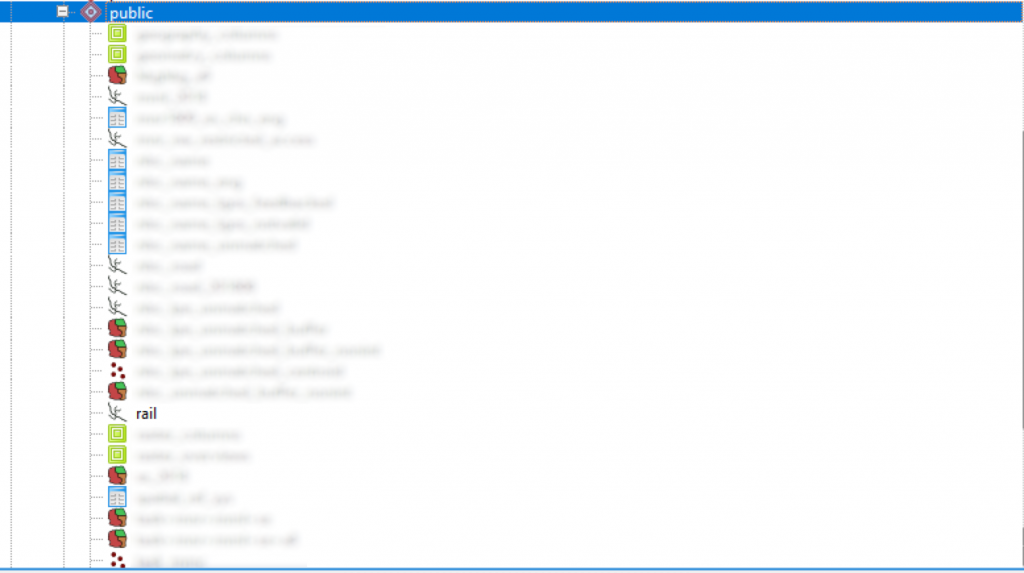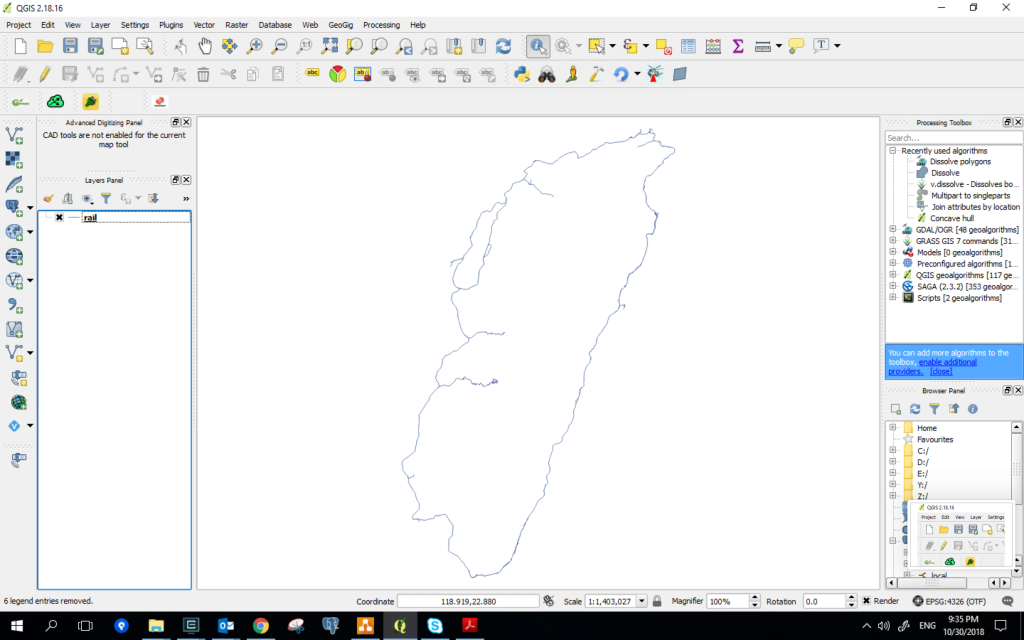``````sql='select * from public.rail '
df = gpd.GeoDataFrame.from_postgis(sql, engine, geom_col='geom' )
df
``````